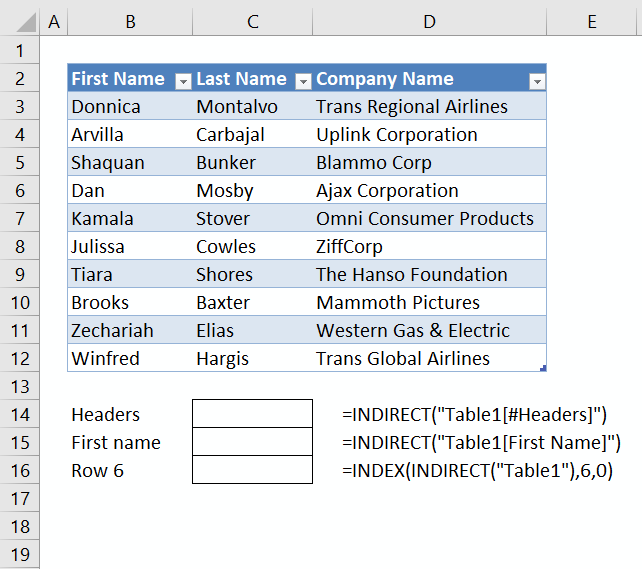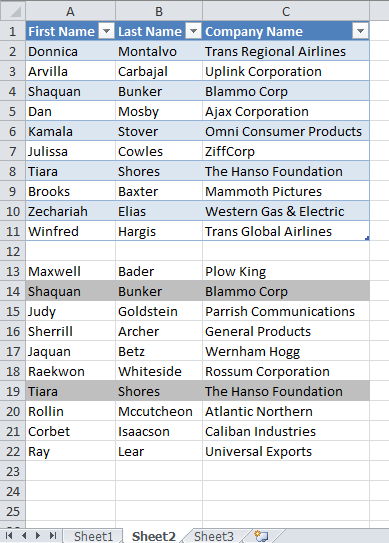Author: Oscar Cronquist Article last updated on February 26, 2018David Hager gave this valuable comment about how to reference a table name in conditional formatting formulas:

=INDIRECT("Table1[Start]")

#### Watch this video to learn more

You can also use this technique in data validation lists. I used it in the three drop down lists below. The formulas are in C13:C15.Reference table headers in a drop down list, cell B13:

Read more about cell references to Excel defined tables (structured references):

Become more productive – Learn Excel Defined Tables

An Excel table allows you to easily sort, filter and sum values in a data set where values are related.

Reference a table column in a drop down list, cell B14:

=INDIRECT("Table1[First Name]")

Recommended article

How to use the INDIRECT function

The INDIRECT function returns the cell reference based on a text string and shows the content of that cell reference. […]

Reference a table row in a drop down list, cell B15:

=INDEX(INDIRECT("Table1"),6,0)

Recommended article:

How to use the INDEX function

Gets a value in a specific cell range based on a row and column number.

Add new items to a drop down list automatically

A drop down list in excel prevents a user from entering an invalid value in a cell. Did you know that you […]

Create dependent drop down lists containing unique distinct values

This article explains how to build dependent drop down lists. Here is a list of order numbers and products. We […]

### Table names in conditional formatting formulas

I used the same technique in the following example:A row is highlighted if a record in cell range A13:C22 is equal to a record in the excel defined table (A1:C11)

Conditional formatting formula, cell range A13:C22:

=COUNTIFS(INDIRECT("Table2[First Name]"),\$A13,INDIRECT("Table2[Last Name]"),\$B13,INDIRECT("Table2[Company Name]"),\$C13)

How to use the COUNTIFS function

Checks multiple conditions against the same number of cell ranges and counts how many times all criteria are met.

Filter shared records from two tables

I will in this blog post demonstrate a formula that extracts common records (shared records) from two data sets in […]

Recommended article:

Highlight closest number

This post demonstrates how to highlight records with the closest value to a criterion, you can also choose to highlight […]